### Taylor Series (Linearization)

The Taylor series (linearization) method is the most commonly used method to estimate the covariance matrix of the regression coefficients for complex survey data. It is the default variance estimation method used by PROC SURVEYLOGISTIC.

Using the notation described in the section Notation, the estimated covariance matrix of model parameters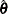by the Taylor series method is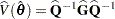where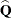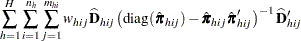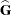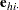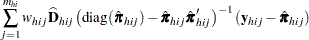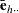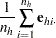and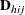is the matrix of partial derivatives of the link function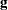with respect toand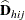and the response probabilities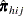are evaluated at.

If you specify the TECHNIQUE=NEWTON option in the MODEL statement to request the Newton-Raphson algorithm, the matrixis replaced by the negative (expected) Hessian matrix when the estimated covariance matrix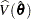is computed.

#### Adjustments to the Variance Estimation

The factor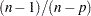in the computation of the matrixreduces the small sample bias associated with using the estimated function to calculate deviations (Morel 1989; Hidiroglou, Fuller, and Hickman 1980). For simple random sampling, this factor contributes to the degrees-of-freedom correction applied to the residual mean square for ordinary least squares in whichparameters are estimated. By default, the procedure uses this adjustment in Taylor series variance estimation. It is equivalent to specifying the VADJUST=DF option in the MODEL statement. If you do not want to use this multiplier in the variance estimation, you can specify the VADJUST=NONE option in the MODEL statement to suppress this factor.

In addition, you can specify the VADJUST=MOREL option to request an adjustment to the variance estimator for the model parameters, introduced by Morel (1989):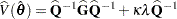where for given nonnegative constants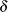and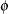,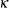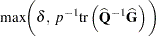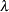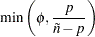The adjustment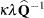does the following:

• reduces the small sample bias reflected in inflated Type I error rates

• guarantees a positive-definite estimated covariance matrix provided that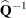exists

• is close to zero when the sample size becomes large

In this adjustment,is an estimate of the design effect, which has been bounded below by the positive constant. You can use DEFFBOUND=in the VADJUST=MOREL option in the MODEL statement to specify this lower bound; by default, the procedure uses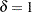. The factorconverges to zero when the sample size becomes large, andhas an upper bound. You can use ADJBOUND=in the VADJUST=MOREL option in the MODEL statement to specify this upper bound; by default, the procedure uses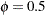.